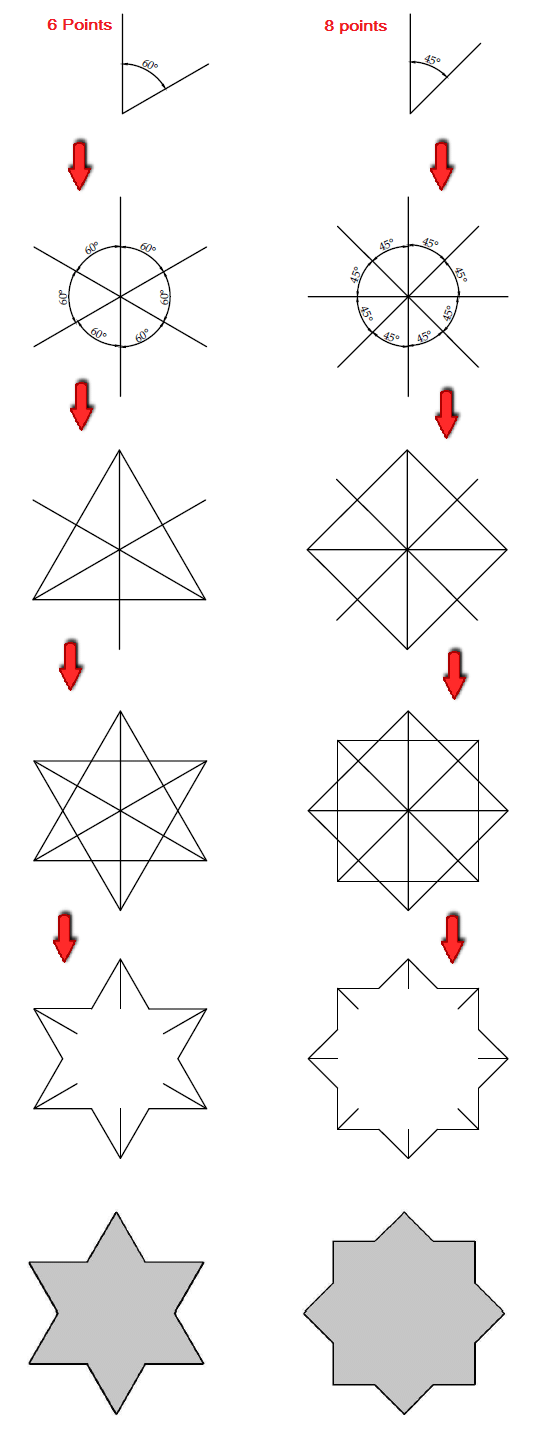How to draw a star

# How to draw a star

This is how to draw a star using a protractor and some common sense facts.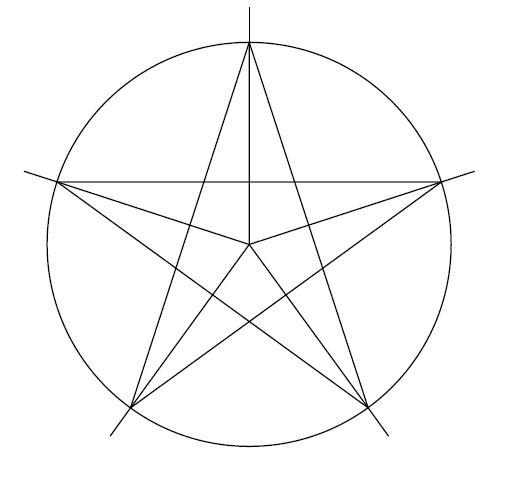## How to draw a star, method 1

A perfect star has all edges uniformly distributed around a circle. Means, if we have a 5 points star with edges A, B, C, D and F, the segment AB, BC, CD, DF and FA are equal.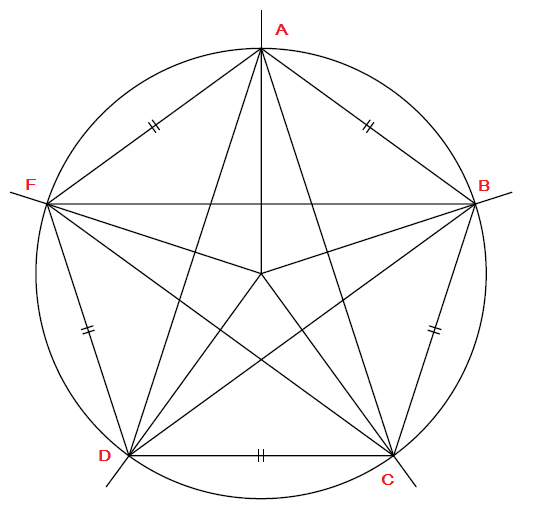This being said and knowing that going from the point A to A around the circle gives us an angle of 360 degrees, if we have 5 edges displaced uniformly around 360 degrees, it means going from the point A to B will be 360 degrees divided by 5 so 72 degrees.

We are going to use this deduction to draw a perfect star.

### Step 1

I need to draw a vertical line of distance x (x being any distance according to the scale of the star you are trying to draw)

### Step 2

Draw a second line with one end common with the line drawn above, and the other end placed 72 degrees away from the other end of the first line. (Use a protractor)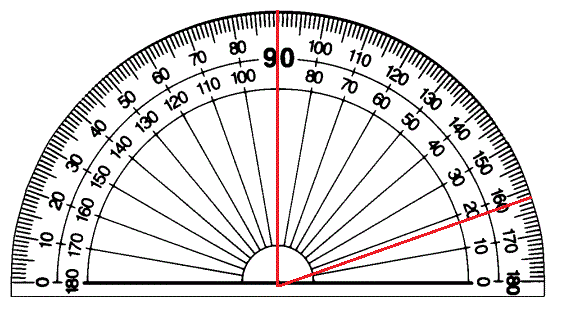Will have them look like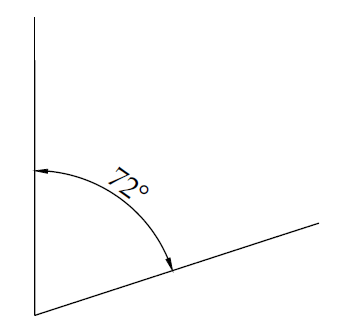### Step 3

Repeat the same process to get other edges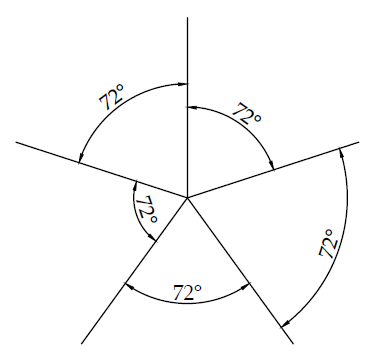### Step 4

Join edges such as shown below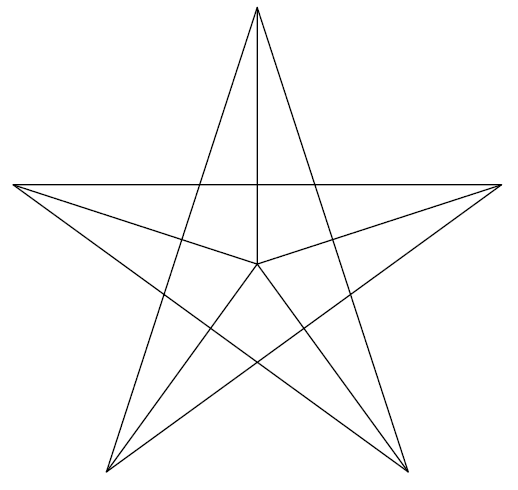Step 5

All you have to do to get a star is to delete unnecessary lines and you have a perfect star.## How to draw a star, method 2

If you would like to use a CAD program to draw a star, check how I used AutoCAD to draw a star.

If you would like to draw a 6 point star, use this same concept, but divide 360 by 6 instead to find proper angles.